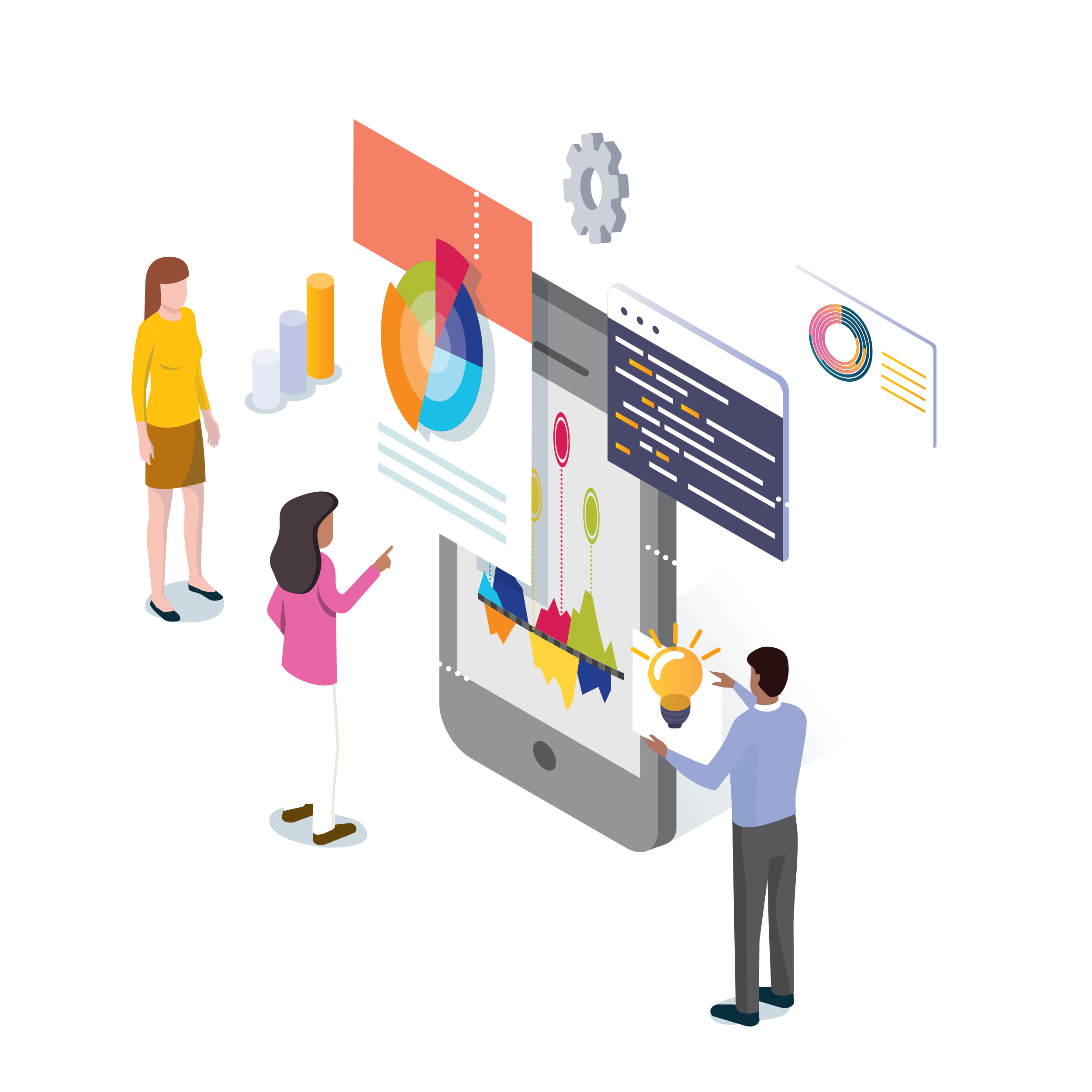MODEL

## Math 7-9Math 7-9 > Factors > Measurement

# Measurement

## Factor Connections

Hover to see how factors connect to Measurement. Then click connected factors to explore strategies related to multiple factors.

How Measurement connects to...

Measurement involves identifying an abstract spatial or temporal component of an entity (e.g., length) and comparing it to a measure unit that has the same attribute (e.g., an inch, a minute) so that the component can be assigned a numerical value, readily reasoned with, and used in calculation. Measurement is the basis for scientific inquiry and provides access to other math concepts, including estimation, fractions, and Statistical Reasoning.

### Main Ideas

Proficiency in Measurement includes:

• Understanding of unit: Knowing that all units must be the same size and that there should be no gaps or overlaps between measure units;
• Basic measurement: Being able to measure a spatial or temporal attribute using repeated standard or informal units (e.g., inches or paperclips; seconds or foot taps);
• Measurement estimation: Being able to approximate a measurable value without the use of measurement tools;
• Numerical reasoning: Being able to use formulas for measurement computations (e.g., area, volume) and converting units; and
• Measurement sense: Understanding how and why measurement works.